New update is available. Click here to update.
Last Updated: Oct 17, 2023
Medium

# Must-do Math for Competitive ProgrammingKushleen Waraich

Competitive Programming as the names suggest is kind of programming in any contest or competition. In other words, it is brain sport which usually takes place over the internet or local network means it’s kind of competition of programming.

Suppose if I make a group of five people to do a particular task with some provided specifications and who will do the task first will get a reward then there would be the atmosphere of competition, everyone will try hard to solve the task than other so he or she could win the task. Competitive Programming is also, same as the situation explained above were in a competition or event programmers take part and solve the problem first then the other than in given time constraint.

Also see, Must Do Coding Questions

## Math for Competitive Programming

As today’s programmers or learners get to know from different resources what Competitive Programming is? It seems very fascinating to them that it would help them to solve problems quicker than other so they jump directly to Competitive Programming but they shouldn’t do this without learning basic data structures. Ultimately they would not be able to learn Competitive Programming and thinks it is not for them as there is a phrase in English “Not knowing and blaming others”. So without learning basic data structure, you would not be able to do competitive programming. You can only do it if you have a really good knowledge of data structures and algorithms.

Now the other thing that comes to mind is why math is important for competitive programming? Then you must know that competitive programming is not any shortcut/trick or magic. In Competitive Programming, various math formula/theorems are used for solving problems to help us get rid of time complexity. Advanced data structures and algorithms are based on math or specifically “DISCRETE MATHEMATICS”. Although it doesn’t require any rocket science or high-level calculus but some intermediate math theorems would be helpful.

There are a number of math theorems which you would require during Competitive Programming but must do are discusses below because of most of the time in a coding problem is based on the following:

Bit Manipulation: It means changing or doing modification with bits. As we know that in C++ integers take 32 bits than in this how can we use those 32 bits for optimising the code? Although it is mostly used in data compression but in competitive programming it will help to optimise code drastically in some problems. One of the most useful and effective low-level optimisations is bit manipulation or using the bits of an integer to represent a set. Not only does it produce an order-of-magnitude improvement in both speed and size, but it can also often simplify code at the same time.

Bitwise Operators:

• NOT ( ~ )
• AND ( & )
• OR ( | )
• XOR ( ^ )
• Left Shift ( << )
• Right Shift ( >> )

Example:

How to generate all possible subset of a set? Suppose you’re given with set A = {1, 2, 3} then no. of subsets should be 2 n -1 which are { 1 }, { 2 }, { 3 }, { 1, 2 } , { 2, 3 }, { 3, 1 }, { 1, 2, 3 }. A big advantage of bit manipulation is that it can help to iterate over all the subsets of an N-element set. As we all know there are 2 N  possible subsets of any given set with N elements.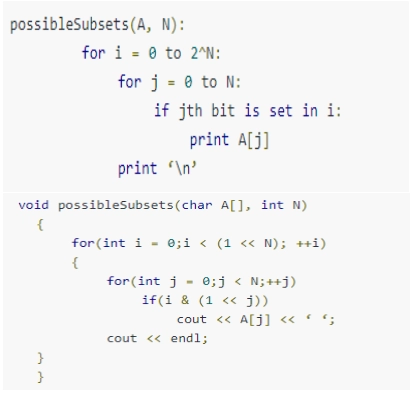Explanation: We need 3 bits for representing all elements 1 represents that the corresponding element is present in the subset, while 0 means that the corresponding element is not there.
0 = (000) 2  = {}
1 = (001) 2  = {3}
2 = (010) 2  = {2}
3 = (011) 2  = {2, 3}
4 = (100) 2  = {1}
5 = (101) 2  = {1, 3}
6 = (110) 2  = {1, 2}
7 = (111) 2  = {1, 2, 3}

BigInteger: For calculating factorials of large numbers which even C++ can’t store in “long long int”. If we think to use Python, which gives us reliability in many of such cases because we can use its “sys.setrecursionlimit( )” upto a fixed number ultimately python will also give an error in it. Another way of doing this in C++ is to use vector and we could use the concept of hashing wisely here, each number will hold an index of an array, e.g. number is 18945 then 18945%10=5 will be stored at index and the number now=18945/10=1234. now 7894%10=4 will be in  and so on to 1%10=1 is in , or you can use string too, it is easier since char array only allow 1 byte for each index so you don’t need that modulation operation to fit number into an index. Java has BigInteger class to hand this.

Modulo Arithmetic: When we divide a number by another number then modulo operation finds the remainder denoted by the % symbol. A mod n means the remainder when a is divided by n
a = qn + r
Theorems which helps us in learning more of Modulo exponential, inverse are Chinese Remainder Theorem, Euler’s Totient Function, Wilson’s Theorem etc.

Properties:

• (a+b)% c= (a%c+b%c)%c
• (a?b)%c = ((a%c)?(b%c))%c
• (a?b)%c = ((a%c)?(b%c)+c)%c
• (a/b)%c = ((a%c)?(d%c))%c

It helps in solving many problems in less time and performs better. Some problems are as follows:

• Multiply large integers under large modulo
• Calculate n! under modulo p
• Modular Multiplicative inverse
• Calculate n C r % p
• Square root % p
• Check if N leaves only distinct remainders on division by all values up to K
• Product of divisors of a number from a given array of its prime factors

Sieve of Eratosthenes and Segmented Sieve

Sieve of Eratosthenes-Time Complexity O(n log long ): It is an ancient algorithm for finding the prime numbers up to the given limit by the following steps:

• Mark the smallest number that is not already visited or checked
• Cross out all the multiples of a number you marked in step 1 except the marked number itself
• You’ve to repeat step 1 and 2 until every number on the table/grid is either visited or crossed out.

Once completed, the marked numbers with which you are left are the primes! Use this algorithm to find all prime number less than 100 then.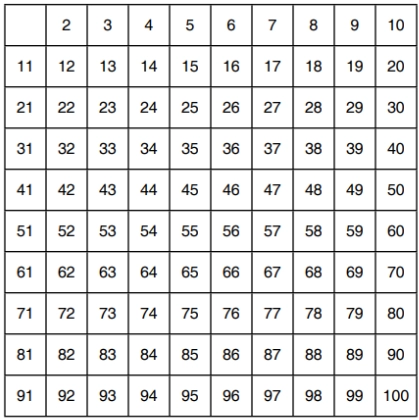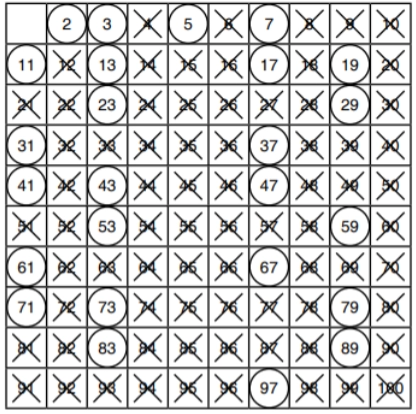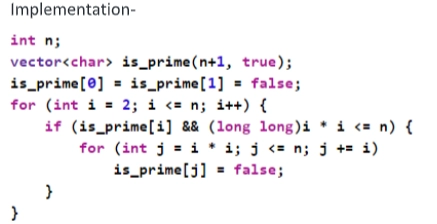Segmented Sieve: It follows from the optimization “sieving till root”. The basic idea of a segmented sieve is to choose the sieving primes less than the square root of n, choose a reasonably large segment size that nevertheless fits in memory, and then sieve each of the segments, in turn, starting with the smallest. At the first segment, the smallest multiple of each sieving prime that is within the segment is calculated, then multiples of the sieving prime are marked as a composite in the normal way; when all the sieving primes have been used, the remaining unmarked numbers in the segment are prime. Then, for the next segment, for each sieving prime, you already know the first multiple in the current segment (it was the multiple that ended the sieving for that prime in the prior segment), so you sieve on each sieving prime and so on until you are finished.

GCD (Euclid Algorithm) and Extended Euclid Algorithm

Euclid Algorithm: As we know GCD of two numbers is the largest number that divides both of them. One way of doing it is just seen the factors of both numbers and multiply the common between them.

Algorithm:

• The first way of doing it if we try to subtract the smallest number from the greatest, GCD remain the same and in this way, if we keep repeating this step we’ll finally get GCD.
• But as above process subtraction could be time-consuming then instead of subtracting what we do, we divide the smaller number, the algorithm stops when we find remainder 0.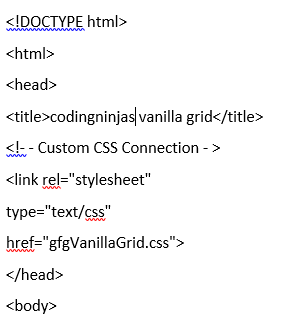Time Complexity: O(log min(a,b))
Extended Euclid Algorithm: It is particularly useful when a and b are coprime while the Euclid algorithm only helps to find out GCD of two numbers while extended Euclid algorithm also tells how to represent the GCD in terms of a and b, i.e. coefficients x and y for which a.x + b.y = gcd(a,b).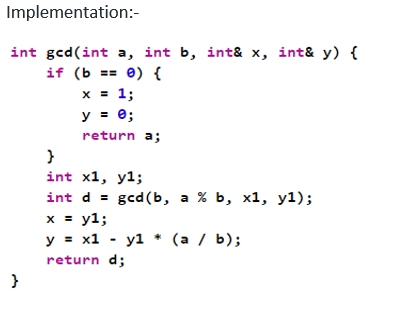Catalan Numbers: These numbers are a sequence of natural numbers that helps in solving many counting problems. Terms starting with n=0 are: 1, 1, 2, 5, 14, 42, 132….and so on. Questions based on Catalan numbers appear in many online competitions. Some of the famous problems are:

• Balanced Parentheses: Suppose you have n pairs of parentheses. For example “()()” is valid but ”(()” is invalid and you have to tell how many groupings are there for each value of n?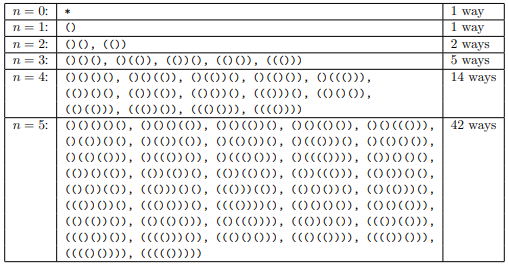• Mountain Ranges: How many mountain ranges can you form with n upstrokes and n downstrokes that all stay above the original line?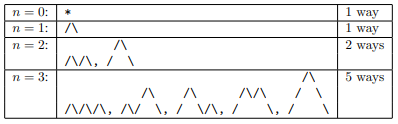• Diagonal-Avoiding Paths: In a grid of n×n squares, how many paths are there of length 2n that lead from the upper left corner to the lower right corner that does not touch the diagonal dotted line from upper left to lower right? In other words, how many paths stay on or above the main diagonal?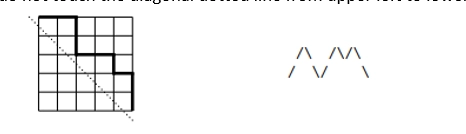• Polygon Triangulation: If you count the number of ways to triangulate a regular polygon with n + 2 sides, you also obtain the Catalan numbers. The figure illustrates the triangulations for polygons having 3, 4, 5 and 6 sides.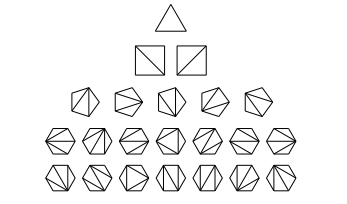• Hands Across a Table: If 2n people are seated around a circular table, in how many ways can all of them be simultaneously shaking hands with another person at the table in such a way that none of the arms crosses each other.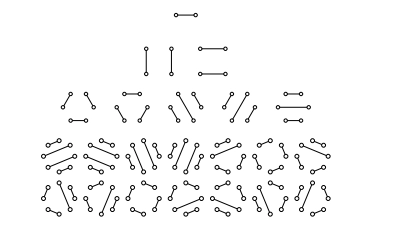• Multiplication Orderings: Suppose you have a set of n + 1 numbers to multiply together, meaning that there are n multiplications to perform. Without changing the order of the numbers themselves, you can multiply the numbers together in many orders.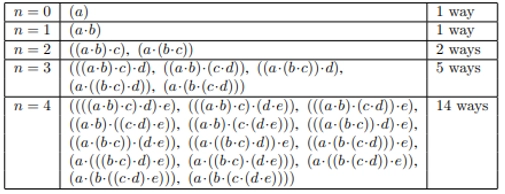• Skew Polyominos: A polyomino is a set of squares connected by their edges. A skew polyomino is a polyomino such that every vertical and horizontal line hits a connected set of squares and such that the successive columns of squares from left to right increase in height—the bottom of the column to the left is always lower or equal to the bottom of the column to the right.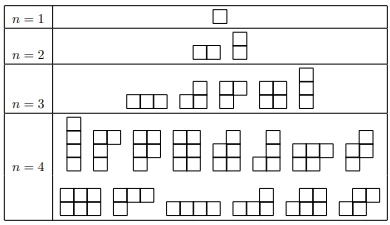• Plane Rooted Trees: A plane rooted tree is just like the binary tree above, except that a node can have any number of sub-nodes; not just two.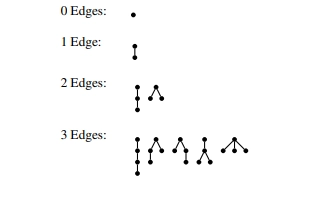• Binary Trees: The Catalan numbers also count the number of rooted binary trees with n internal nodes. Illustrated in Figure 4 are the trees corresponding to 0 ≤ n ≤ 3. There are 1, 1, 2, and 5 of them. Try to draw the 14 trees with n = 4 internal nodes.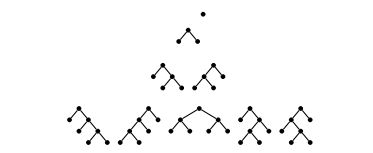Primality Test: It is an elementary method for finding if a given number is prime so what is our basic approach to find a number as we have already studied till elementary school. If a number is divisible by itself and 1 only then that number is said to be prime and if we have to make a program on this then what we generally do we iterate in a loop from 2 to n-1 then see if that number is divided between any number in between if it is divisible then it is nonprime otherwise it is prime. This a good approach and will also work giving complexity of O(n) but we can optimise it for very large input.

Optimised Code:

• As we were checking till all numbers from 2 to n-1, we can check till √n because a larger factor of n must be a multiple of smaller factor that has been already checked.
• Another way is if we observe all prime numbers then we see that all primes are of the form 6k ± 1, other than 2 and 3. So a more efficient method is to test if n is divisible by 2 or 3, then to check through all the numbers of form 6k ± 1.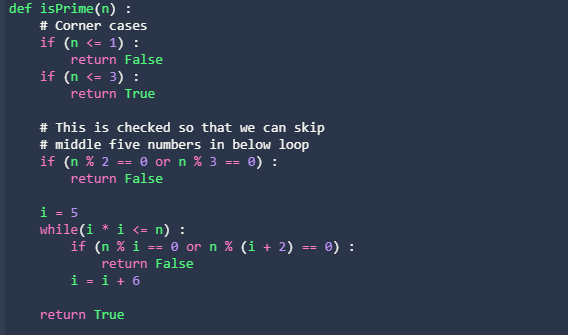Although there is no. of Other algorithms/theorems but important are discussed above. Others are:

• Fermat’s theorem, Euler Totient theorem ( totient function, order, primitive roots)
• Chinese remainder theorem
• Integer Factorization (Pollard Rho factorization)
• Logarithmic Exponentiation
• Stirling numbers
• Wilson Theorem
• Lucas Theorem

1. Basic probability and Conditional probability
2. Random variables, probability generating functions
3. Bernoulli, Binomial, Poisson, normal distribution
4. Counting
5. Basic principles – Pigeon hole principle, addition, multiplication rules
6. Inclusion-exclusion
7. Stirling, eulerian, harmonic, Bernoulli, Fibonacci numbers
8. Advanced counting techniques – Polya counting, burnsides lemma

You can also consider our competitive programming course to give your career an edge over others!